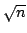Statistica Sinica 20 (2010), 513-536

A SEMIPARAMETRIC APPROACH

FOR A MULTIVARIATE SAMPLE SELECTION MODEL

Marie Chavent, Benoît Liquet and Jérôme Saracco

University of Bordeaux

Abstract: Most of the common estimation methods for sample selection models rely heavily on parametric and normality assumptions. We consider in this paper a multivariate semiparametric sample selection model and develop a geometric approach to the estimation of the slope vectors in the outcome equation and in the selection equation. Contrary to most existing methods, we deal symmetrically with both slope vectors. Moreover, the estimation method is link-free and distribution-free. It works in two main steps: a multivariate sliced inverse regression step, and a canonical analysis step. We establish-consistency and asymptotic normality of the estimates. We describe how to estimate the observation and selection link functions. The theory is illustrated with a simulation study.

Key words and phrases: Canonical analysis, eigen-decomposition, multivariate SIR, semiparametric regression models, sliced inverse regression (SIR).